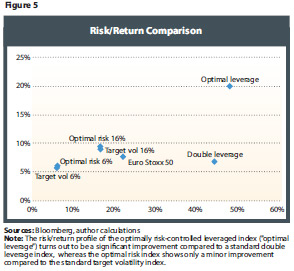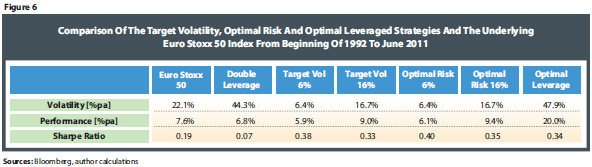Optimal Design Of Risk Control Strategy Indexes

August 17, 2012

We simulate a constant leverage strategy (3) with L=2, the target volatility strategy (6), the optimally risk-controlled leveraged strategy (7) (which we refer to as "optimal leverage") and the optimal target volatility strategy (8) (referred to as "optimal risk") using the Euro Stoxx 50 as an underlying equity investment from 1992 to 2011. For the target volatility strategy and the optimal risk strategy, we run two simulations—one with a target volatility level of 6 percent and one with 16 percent. The performance is plotted in Figure 4, whereas the risk/return matrix is shown in Figure 5. Figure 6 summarizes the key performance figures and risk/return characteristics.We can draw two key observations from Figures 4 and 5. The first is that the target volatility strategy is very close to the optimal risk strategy in terms of risk/return profile and can therefore be considered as a very good proxy for the optimal risk investment scheme. Secondly, except for the constant leverage strategy, all schemes have a better risk/return profile than the underlying Euro Stoxx 50 Index, including the optimally risk-controlled leverage scheme ("optimal leverage"), which shows an impressive outperformance over 19 years.

The most popular investment scheme in the retail market (especially in the form of leveraged ETFs) is the double leverage approach, which shows a poor performance and very high levels of volatility in the long run. We therefore argue that investors are better off investing for the long term in either the optimal leverage strategy or the target volatility scheme, depending on their risk appetite.